Zhang, X., Li, J., Zhang, Y., Cai, W., and Liu, H. (2016). "Data-driven method for pulp properties estimation in stock preparation," BioRes. 11(2), 4947-4963.

#### Abstract

Pulp properties are key factors to assessing the performance of a refining process, evaluating operational conditions, and optimizing the process of stock preparation. This paper presents a data-driven approach to estimate the beating degree and wet weight of pulp after refining using case-based reasoning (CBR). Historical data generated in a refining process at a paper mill was used to evaluate the proposed model. The root mean square error (RMSE) and coefficient of variance of the root mean square error (CV-RMSE) of the beating degree estimation results in CBR were 1.30 and 4.32%, respectively, and the RMSE and CV-RMSE of the wet weight were 0.50 and 19.09%, respectively. The results of beating degree prediction were satisfactory, and the results of wet weight were also acceptable. To test the performance of CBR model, support vector machine algorithm (SVM) were employed to verify the effectiveness and accuracy. The RMSE and CV-RMSE of the beating degree estimation results in SVM were 1.20 and 4.02%, respectively, and the RMSE and CV-RMSE of the wet weight were 0.44 and 16.73%, respectively. As a result, the proposed model was as accurate as the SVM method.

Data-driven Method for Pulp Properties Estimation in Stock Preparation

Xiangyu Zhang, Jigeng Li,* Yanzhong Zhang, Wei Cai, and Huanbin Liu

Pulp properties are key factors to assessing the performance of a refining process, evaluating operational conditions, and optimizing the process of stock preparation. This paper presents a data-driven approach to estimate the beating degree and wet weight of pulp after refining using case-based reasoning (CBR). Historical data generated in a refining process at a paper mill was used to evaluate the proposed model. The root mean square error (RMSE) and coefficient of variance of the root mean square error (CV-RMSE) of the beating degree estimation results in CBR were 1.30 and 4.32%, respectively, and the RMSE and CV-RMSE of the wet weight were 0.50 and 19.09%, respectively. The results of beating degree prediction were satisfactory, and the results of wet weight were also acceptable. To test the performance of CBR model, support vector machine algorithm (SVM) were employed to verify the effectiveness and accuracy. The RMSE and CV-RMSE of the beating degree estimation results in SVM were 1.20 and 4.02%, respectively, and the RMSE and CV-RMSE of the wet weight were 0.44 and 16.73%, respectively. As a result, the proposed model was as accurate as the SVM method.

Keywords: Stock preparation; Pulp quality; Data-driven model; Case-based reasoning

Contact information: State Key Laboratory of Pulp and Paper Engineering, South China University of Technology, Guangzhou 510640, P.R. China; *Corresponding author: jigengli@scut.edu.cn

INTRODUCTION

Mechanical treatment influences various pulp properties such as freeness, fiber length, water retention value, specific surface area, surface charge, etc., when pulp fibers are being refined or beat in a refiner (Fardim and Durán 2003; Bhardwaj et al. 2007a, 2007b; Banavath et al. 2011; Gharehkhani et al. 2015). Generally, the PFI refiner and Valley beater are more often used in the laboratory, and conical refiner and disc refiner are used in industrial settings.

Refining is one of the most important unit operations in paper manufacturing, as it enhances the paper sheet properties by creating external fibrillation and internal delamination, and increasing the development of specific surface area (Stoere et al. 2001; Gharehkhani et al. 2015). The Shopper-Riegler degree is a well-known pulp drainability measurement, and paper sheet properties are generally presented as a function of the Shopper-Riegler value (°SR) (Helmerius et al. 2010). In addition, fiber length correlates to sheet strength and tear index and strongly influences paper formation (Cowan 1995). The mechanical treatment of cellulose fibers can be directly applied to biochemical conversion processes to overcome biomass recalcitrance to enzyme hydrolysis and increase the lignocellulosic biomass enzymatic digestibility by opening up the biomass structure to increase the cellulose accessibility to enzymes. The water retention value is a good predictor of improvements in sugar conversion for a given fiber source and composition (Jones et al. 2014; Park et al. 2016). Xu et al. (2014) found that the beating degree of PFI-refined substrates had a strong linear correlation with final total sugar yields, such that the final sugar yield could be predicted by the beating degree of PFI-refined corn stover. Namely, the refined fiber properties are closely related to the end-use properties. Therefore, a model for estimation of pulp properties based on operational variables is an important tool for operators to evaluate and optimize the process.

The effects of refining on fibers have been investigated thoroughly, with many theories explaining the relationship between refining action and its results (Miles and Karnis 1991; Baker 1995; Fardim and Durán 2003; Gharehkhani et al. 2015). These models have clarified the refining process, and mechanistic and empirical models have been used to predict the properties of refined pulp (Olson et al. 2003). In addition, linear regression models, multiple regression analysis, and neural fuzzy models are often employed to examine the influence of operation variables on pulp and paper sheet properties and to identify the optimum operating conditions of pulping and refining (Broderick et al. 1997; Ferrer et al. 2011; González et al. 2013). When studying the impact of refining operations on pulp quality, multivariate analyses (MVA) including the partial least squares (PLS) method establish relationships between the experimental data and the fiber properties (Broderick et al. 1996; Bhardwaj et al. 2007a, 2007b).

As a new reasoning technique and machine-learning method in artificial intelligence (AI), case-based reasoning (CBR) models imitate human reasoning, using specific knowledge collected on previously encountered situations to solve new situations. This method has the advantage of gaining knowledge conveniently with high efficiency, and it does not require transcendental knowledge and other related information. The CBR method was usually used in mechanical engineering, medicine, business administration etc. for classification, diagnosis, and decision support (Lenz et al. 1998). Besides, the CBR model also could be employed for prediction in process industry. These models were developed using historical process data and on-line properties or lab test measurements. In addition, the computation complexity is only linear with respect to the number of attributes and cases (Zhou and Chai 2014; Han and Cao 2015; Yan et al.2015).

In pulping and refining processes, there is a common view that operating conditions can be duplicated. When the same operation conditions are applied in another trial with the same installations, it is no surprise that the resultant pulp properties are the same. In this study, CBR method was used to develop a model for estimating pulp properties. Industry experiments were conducted in a stock preparation refining process, and the results show the effectiveness of this method.

EXPERIMENTAL

Model Development Method

Case-based reasoning

The quality of pulp greatly depends on operating conditions. For a given refiner installation, the same operational conditions produce pulp with consistent properties. The case-based reasoning estimation of pulp properties in this paper is based on the concept that operating conditions can be duplicated. Namely, the current pulp properties can be predicted from the properties of pulp generated previously in similar conditions.

In general, the CBR modeling process mainly includes case representation, case retrieval, case reuse, case revision, and case retention (Zhou and Chai 2014; Yan et al. 2015). The strategy diagram of the CBR modeling method is shown in Fig. 1.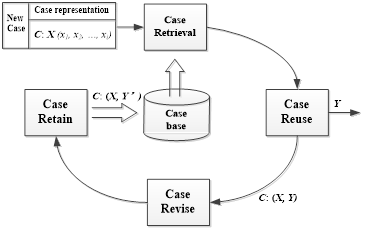Fig. 1. Strategy diagram of the CBR soft-sensor for pulp quality

Case representation

In the first step—case representation—the past operating conditions are arranged with corresponding pulp properties in cases with suitable form and then stored in the case base.

For cases, the operational variables X (x1, x2, …, xi) (i = 1,2, …, k) were condition features, and the solution Y was the pulp quality value. Suppose each case Cn (n = 1, 2, …, p) was expressed in the following pair-wise functions,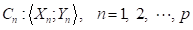(1)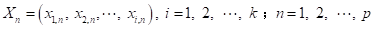(2)

where p is the total number of the cases in case base, k is the number of the operational variables, and Xn and Yn denote the operational variables and the solution (pulp property), respectively, in the nth cases. The variables xi,n denote ith condition features of nth cases in the case base.

Case retrieval and case reuse

When pulp properties in new operational conditions need to be estimated, the conditions of the new case (C: X without Y) are searched in the case base to find similar past cases. The pulp property (Y) corresponding to the present case is estimated using properties stored in similar past cases.

To find similar cases, the similarity (SIM) of the new case (x1, x2, …, xi) with each past case is calculated. A larger SIM value indicates greater similarity between cases. The simi value is the similarity of ith condition feature (xi) of new and past cases (Eq. 3):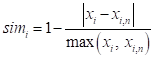(3)

Afterwards, the similarity (SIMn) of the new case C(x1, x2, …, xi) with nth past case (Cn) can be obtained as follows,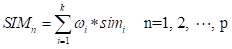(4)

where ωi (i = 1, 2, …, k) is the weight of the ith condition feature attribute, representing the degree to which the similarity (simi) of a condition feature could affect the SIMn result of new case and past cases. The constraint condition is: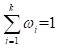and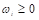(5)

The p similarities (SIM) are obtained by Eq. 4 and ranked from small to large. Conventionally, the K-nearest neighbor (K-NN) method is employed to determine which cases are most similar, where K is the number of neighbors and Y1 through YK are K case solutions corresponding to the top K similarities (SIM). The unknown outcome, Y, of a new case can then be obtained by calculating the mean value of the K case solutions (Eq. 6):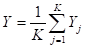(6)

However, the conventional K-NN method is highly influenced by the value of K. If K is too high, the CBR retrieves too many unrelated cases, which may lead to poor results. In contrast, if K is too small, the CBR may lack sufficient reference cases to make a correct decision. In this paper, the estimated value Y is not determined using the constant K cases, and instead the neighbor cases were retrieved if their SIM was equal to or greater than a threshold value (SIMTV) (Zhou and Chai 2014). The threshold (SIMTV) is a given value and was determined in the CBR process as follows: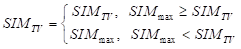(7)

where SIMmax = Max (SIMn), n = 1, 2, …, p.

Then, the new case solution was obtained as follows: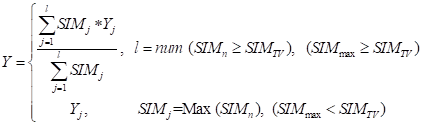(8)

where l was the number of cases where the SIM was equal to or greater than SIMTV and SIMj is the jth SIM. The solution Y was used as the final result for output.

Case revision and case retention

If there are enough cases in the case base covering all possible process conditions, a satisfying solution could be obtained after the case reuse phase. For incremental learning, it is important to study how to evaluate and revise the reuse results and add the revised new cases to the case base.

At first, it is impossible to exactly match new cases and past cases. If the estimated value is in the specification limitation or is a correct result, as evaluated by expert operators, the new case C(X; Y) (with the new solution Y) is stored in the case base. When the result generated by the case reuse is not correct, it is more likely to learn failure. There are two solutions: drop this case confirmed by experts or introduce the correct data according to offline data and store it in the case base, as shown in Fig. 2.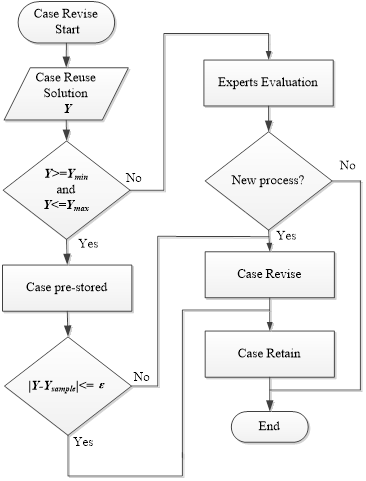Fig. 2. Flow diagram of case revise and case retain process

When the next process data emerges, the pulp quality can be monitored in real time by repeating the process above. Moreover, as the number of cases increases, the case base expands, and the efficiency of case retrieval is affected. Therefore, cases with high similarity should be reduced after screening to control the scale of database.

The Refining Process in Stock Preparation

In a typical stock preparation, virgin pulp from commercial bales is slushed and screened before refining. The pulp is pumped into a refiner feed chest to keep stock consistency and flow rate constant at different levels for different production plans.

In this study, the raw pulp was a blend of bleached softwood kraft pulp (NBKP) and bleached eucalypt kraft pulp (LBKP). They were mixed together in the pulper before refining. In the main line, pulp is refined in a series of three cascading refiners. Pulp consistency was measured before refining, and the pulp flow rate was measured after the third refiner as shown in Fig. 3.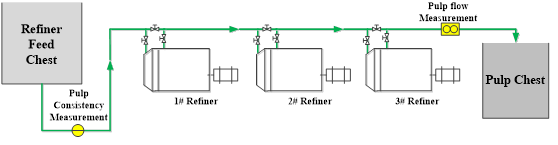Fig. 3. Flow chart of the refining process in stock preparation

The most common theories to describe the refining process are the specific edge load (SEL), the specific surface load (SSL), and C-factor theory (Gharehkhani et al. 2015). Although the C-factor theory includes all refining process variables and both the plate and fiber characteristics, SEL and SSL theories are user friendly and popular in practice (Kirby 1980; Baker 1995; Olejnik 2013).

In these theories, many variables affect refining. In industrial sites, however, the refiner plate material and plate pattern configurations do not change, and the motor rotational speed is fixed. The type of refining is predetermined according to the chosen plate and refiner design. The pulp properties are controlled by regulating operating conditions such as refiner load, flow rate, consistency, and pH. Changes in the proportion of NBKP and LBKP in the pulper also affect refining.

The primary variable controlling refining is the specific energy consumption (SEC) (Olejnik 2013), given by Eq. 9,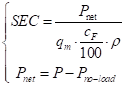(9)

where P is total power consumption in refining process, Pno-load is power to overcome the hydraulic, pumping, and mechanical losses, Pnet is the net power transferred to stock (kW), qm is the pulp flow through refiner (m3/h), cF is the consistency of refined stock (%), and ρ is the pulp density (ton/m3).

Data Collection

Operational variables

Historical data from Zhuhai S.E.Z. Hongta Renheng Paper Co., Ltd. (Zhuhai, China) were used to evaluate the proposed CBR pulp property prediction model, where plant tests were carried out in the stock preparation refining process. The data covered the period of December 2014 to April 2015 and consisted of refining operational variables with corresponding pulp properties.

The retention time of pulp flocs in the refiner is related to pulp consistency (Miles and Karnis 1991), and it affects the number of impacts on pulp fibers conducted by C-factor theory (Gharehkhani et al. 2015). Pulp consistency and flow rate also have a strong influence in industrial operations (Eq. 10). In general, the mill control strategy involves measuring and stabilizing flow rates and consistency for proper process operations, then adjusting applied power to react to process upsets or furnish changes.

The collected operational variable values include the motor power of three refiners, the pulp consistency, flow rate, and pH (Table 1).

Table 1. Operational Variables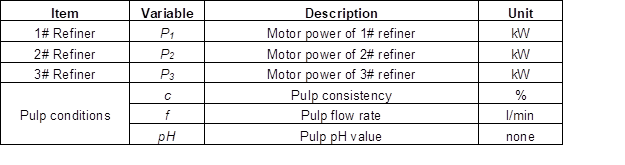Pulp properties

The two most important properties that define the refining pulp are drainage time and fiber length in stock preparation. The Schopper Riegler value (°SR, known as beating degree) is a well-known characterization for drainage time of refined pulp in stock preparation. In an industrial site, wet weight (g) of pulp usually serves as an indirect index of the average fiber length.

In this study, the CBR models were developed to predict beating degree (Y1) and wet weight (Y2) of pulp. The beating degree of pulp was measured using a Schopper Riegler device according to the ISO 5267-1 (1999). Pulp wet weight measurement is determined by the Schopper Riegler apparatus with an accessory which is usually called pulp wet weight framework. Before the wet weight measurement, the weight of framework (g1) is an average value obtained by multiple measurements. When the framework is placed into the drainage chamber, the operating steps of wet weight measurement are the same as the beating degree. Afterwards, the weight of framework and remaining fiber (g2) was determined. The pulp wet weight (g) is equal to g2 minus g1.

Another important factor is the beating degree of pulp when entering the refiner. The beating degree of virgin pulp (y0) was collected before refining.

Model Implementation and Case Learning

To test the performance of CBR model in estimating pulp properties, Matlab 2010b software (MathWorks, Natick, MA, USA) was utilized to build CBR models, and Microsoft Excel 2010 software (Microsoft, Seattle, WA, USA) was used to store the data in the case base. The collected data were stored in groups and arranged by time (Table 2).

Table 2. Experimental Data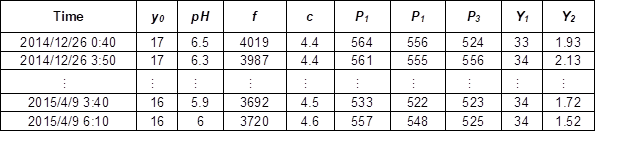The first 150 groups were used as the initial case base. The remaining data was divided into two parts: 100 groups were randomly selected as test data set, and the others were used for case revision and retention, i.e., case learning.

In the case revise and retain process (Fig. 2), the case similarity threshold SIMTV was set to 0.85, and ε was set to 2. Ymin and Ymax were obtained by Eq. 10 as follows,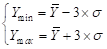(10)

where Y-bar was the mean value of beating degree or wet weight of collected data and σ was the standard deviation of beating degree or wet weight.

There were 290 cases for beating degree and 286 cases for wet weight in the case revise and retain process.

Evaluation Criteria

The performance of the proposed method was evaluated using the root mean square error (RMSE) and coefficient of variance of the root mean square error (CV-RMSE) as defined by Eqs. 11 and 12, respectively,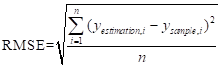(11)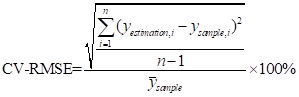(12)

where yestimation,i was the predicted pulp property value, ysample,i was the sample value of the pulp property, ysample-bar was the mean value of sample pulp properties in test set, and n was the number of records of sample in the test set.

RESULTS AND DISCUSSION

Data Analysis and Weight Value ωi Allocation

After removing dubious periods of the operation such as a low production rate and aberrant process behavior data, correlations between the pulp properties and operational variables were investigated. The Pearson correlation coefficient was used to show the association between two variables (U, V) (Eq. 13),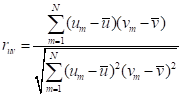(13)

where u-bar and v-bar are the sample mean of U and V. The correlation coefficients of beating degree and wet weight of pulp with the operational variable are listed in Table 3.

Values of a correlation coefficient are always between -1 and +1. A positive value indicates that two variables increase or decrease together; a negative correlation coefficient indicates that as one variable increases, the other decreases. A correlation coefficient of zero indicates that there is no linear relationship between the two variables.

Table 3. Correlation Coefficients of Beating Degree and Wet Weight of Pulp With Operational VariableThe weight value ωi could be obtained by expert or operator knowledge, but this way depends on personal experience and is not objective. Rough set theory and genetic algorithm are usually employed to find the optimum weights (Louhi-Kultanen et al. 2009; Zhou and Chai 2014; Yan et al. 2015). In this study, the Pearson correlation coefficient, which partly recognizes relationships between operational variables and pulp properties, was used to approximate the attribute weight value, ωi, and was obtained as follows,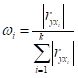(14)

wherewas the correlation coefficient of the pulp quality of the ith operational variable.

The weight values of each operational variable for beating degree and wet weight prediction are listed in Table 4.

Table 4. Weight Values for each Operational Variable for Beating Degree and Wet Weight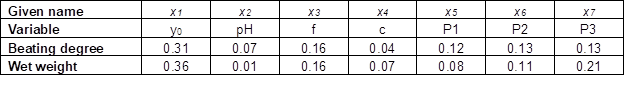Results of the CBR Models

During the beating degree and wet weight estimation process, the case similarity threshold SIMTVwas set to 0.9.

Figure 4 shows the results of beating degree estimation before and after case learning. Figures 4a and 4b are estimation results before and after case learning and the sample values, respectively. Figures 4c and 4d are the scatter diagram of beating degree estimation before case learning and after case learning. As the scatter diagram shows in Fig. 4, the results after case learning were more accurate than before learning. The RMSE and CV-RMSE of the estimation results before case learning were 2.57 and 8.57%, respectively, which decreased to 1.30 and 4.32%, respectively, after case learning. For a direct comparison, the estimated data before and after case learning and the sample values were plotted together in Fig. 5a. The absolute values of the errors between estimation results before and after case learning and the sample values were plotted in Fig. 5b.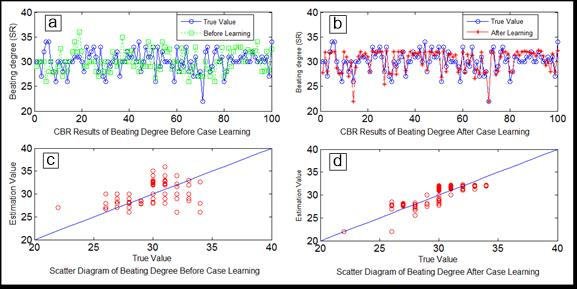Fig. 4. Beating degree estimation results before and after case learning. (a) CBR results of beating degree before case learning; (b) CBR results of beating degree after case learning; (c) scatter diagram of beating degree before case learning; (d) scatter diagram of beating degree before case learning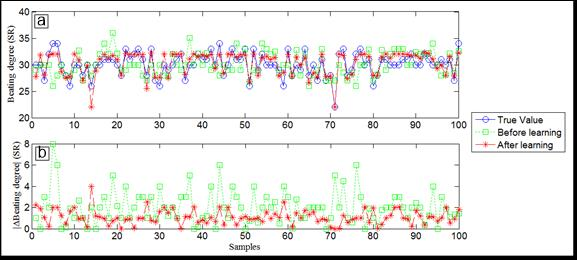Fig. 5.Contrasting results of beating degree before case learning and after case learning. (a) the CBR estimated data before and after case learning and the sample values; (b) absolute values of the errors between estimation results before and after case learning and the sample values

Figure 6 shows the wet weight estimation before and after case learning. Figures 6a and 6b are estimation results before and after case learning and the sample values. Figures 6c and 6d are the scatter diagram of wet weight estimation before and after case learning. As the scatter diagram shows in Fig. 6, the results after case learning were better than before learning. The RMSE and CV-RMSE of the wet weight estimation results before case learning were 1.39 and 52.47%, respectively, which decreased to 0.50 and 19.09%, respectively, after case learning. To directly compare the results, the estimated results before and after case learning and the sample values were plotted in the same coordinate plane as shown in Fig. 7a. The absolute values of the errors between estimation results before and after case learning and the sample values are plotted in Fig. 7b.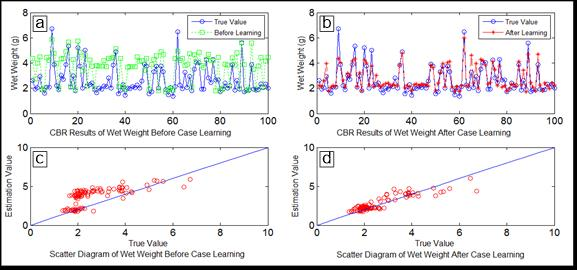Fig. 6. Wet weight estimation results before and after case learning. (a) CBR results of wet weight before case learning; (b) CBR results of wet weight after case learning; (c) scatter diagram of wet weight before case learning; (d) scatter diagram of wet weight before case learning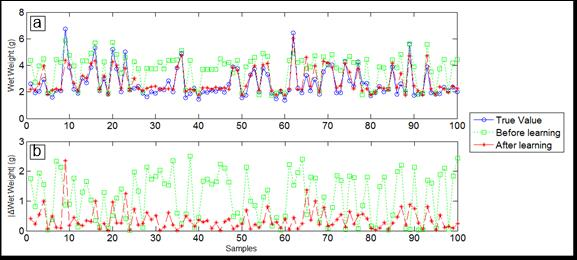Fig. 7. Contrasting results of wet weight before case learning and after case learning. (a) CBR estimated data before and after case learning and the sample values; (b) absolute values of the errors between estimation results before and after case learning and the sample values

Incomplete Case Estimation

In practice, some operational variable values are often missing because mill site instruments fail due to aging, lack of maintenance, and bad operating environment. These situations result in incomplete cases where some conditions are missing. To deal with these problems, the similarity (SIMn) of the new case C(x1, x2, …, xi) with nth past case (Cn) can be updated as follows: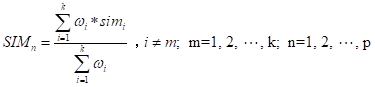(15)

When the mth condition feature is missing in the new case, ωm is set to zero, and simm is not included in the CBR calculation. All the p SIMes can be obtained so that the CBR process could continue.

To test the updated CBR model performance, some condition feature values in the 100 test groups set were deleted randomly. Afterwards, another CBR estimation process of beating degree and wet weight were conducted based on the case base after learning.

Figure 8 shows the contrasting results of beating degree estimation with or without incomplete cases. Figure 8a shows the contrasts between estimation results without and with incomplete cases and the sample values. Figure 8b shows the absolute values of the errors between estimation results without and with incomplete cases and the sample values. The RMSE and CV-RMSE of the beating degree estimation results without incomplete cases were 1.30 and 4.32%, respectively; with incomplete cases, the RMSE and CV-RMSE were 1.73 and 5.79%, respectively. Obviously, the condition feature missing has little influence on the accuracy of CBR prediction results of beating degree.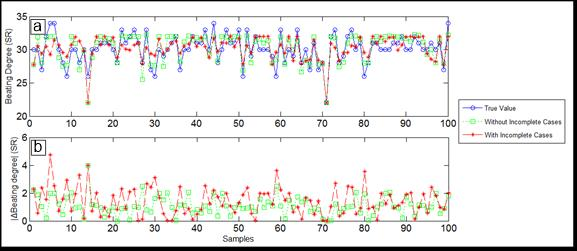Fig. 8. Contrasting results of beating degree estimation without and with incomplete cases. (a) CBR estimated data without and with incomplete cases and the sample values; (b) absolute values of the errors between estimation results without and incomplete with cases and the sample values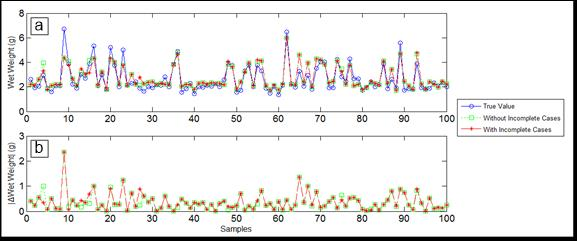Fig. 9.Contrasting results of wet weight estimation without and with incomplete cases. (a) CBR estimated data without and with incomplete cases and the sample values; (b) absolute values of the errors between estimation results without and with incomplete cases and the sample values

Figure 9 shows the contrasting results of wet weight estimation without incomplete cases and with incomplete cases. Figure 9a shows the contrasts between estimation results without and with incomplete cases and the sample values. Figure 9b shows the absolute values of the errors between estimation results without and with incomplete cases and the sample values. The RMSE and CV-RMSE of the wet weight estimation results without incomplete cases are 0.50 and 19.09%, respectively, and the RMSE and CV-RMSE with incomplete cases are 0.51 and 19.23%, respectively. Likewise, the condition feature missing had little influence on the accuracy of CBR prediction results of wet weight. However, in practice, incomplete cases should not be added into the case base of CBR.

Contrasting Results with Different Methods

To test the performance of the CBR model for the estimation of pulp properties, a support vector machine algorithm (SVM) method was used to predict the pulp properties (Kadlec et al. 2009). In the SVM algorithm, the penalty factor was 1, and the kernel function was the Gaussian radial basis function. The width was 2.8284 for beating degree estimation. For wet weight estimation, the penalty factor was 1.4142, the kernel function was the Gaussian radial basis, and the width was 1. The algorithms were designed in Matlab 2010b software.

Figure 10 shows contrasting results obtained due to SVM and CBR of beating degree estimation. Figure 10a shows the contrasts between estimation results of different methods and the sample values. Figure 10b shows the absolute values of the errors between estimation results of different methods and the sample values. The RMSE and CV-RMSE of the beating degree estimation results in SVM were 1.26 and 4.03%, respectively, and the RMSE and CV-RMSE of the beating degree estimation results in CBR were 1.30 and 4.32%, respectively. Figure 11 shows the contrasting results obtained due to SVM and CBR of wet weight estimation. Figure 11a indicates the contrasts between estimation results of different methods and the sample values. Figure 11b portrays the absolute values of the errors between estimation results of different methods and the sample values. The RMSE and CV-RMSE of the wet weight estimation results in SVM were 0.44 and 16.73%, respectively, and the RMSE and CV-RMSE of the wet weight estimation results in CBR were 0.50 and 19.09%. There was not much difference between CBR and SVM.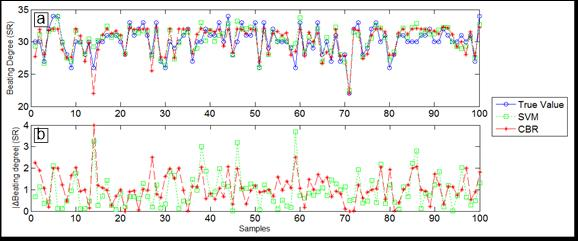Fig. 10. Contrasting results of different methods for beating degree estimation. (a) Estimated data in SVM and CBR and the sample values; (b) absolute values of the errors between estimation results in SVM and CBR and the sample values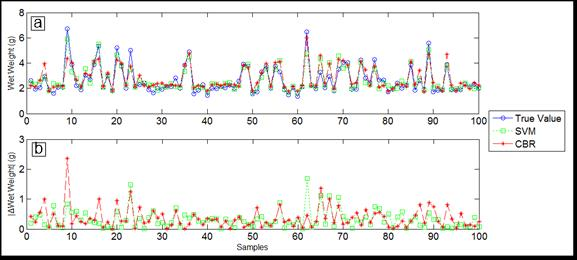Fig. 11. Contrasting results of different methods for wet weight estimation. (a) Estimated data in SVM and CBR and the sample values; (b) absolute values of the errors between estimation results in SVM and CBR and the sample values

CONCLUSIONS

1. In this paper, a data-driven method based on case-based reasoning (CBR) was used to estimate the beating degree and wet weight after refining in stock preparation. The model was evaluated using simulated data collected from a paper mill. The estimation results provided by CBR model concurred with sample tests. The results evaluated by root mean square error (RMSE) and the corresponding coefficient of variance (CV-RMSE) verify the effectiveness of the CBR method.
2. The support vector machine algorithm (SVM) method was used to evaluate the performance of the CBR model. The RMSE and CV-RMSE of the beating degree and the wet weight estimation results in the CBR method are close to the SVM method. The CBR model was as effective as the SVM method in predicting beating degree and wet weight.
3. As an intelligent algorithm, CBR does not need much parameter adjustment during the process of problem solving. When the attribute weight values ωi were determined, the computation complexity was less than SVM. With increased CBR model service time, the prediction will be more precise and accurate. In addition, the CBR model could handle cases with incomplete information. Thus, the method recommended in this paper has practical applications and advantages.

ACKNOWLEDGMENTS

The authors are grateful for financial support from the National Natural Science Foundation of China, Grant. No.61333007, and the help of the engineers at Zhuhai S.E.Z. Hongta Renheng Paper Co., Ltd. (China).

REFERENCES CITED

Baker, C. F. (1995). “Good practice for refining the types of fiber found in modern paper furnishes,” TAPPI J. 78(2), 147-153.

Banavath, H. N., Bhardwaj, N. K., and Ray, A. K. (2011). “A comparative study of the effect of refining on charge of various pulps,” Bioresource Technol. 102(6), 4544-4551. DOI: 10.1016/j.biortech.2010.12.109

Bhardwaj, N. K., Hoang, V., and Nguyen, K. L. (2007a). “Effect of refining on pulp surface charge accessible to polydadmac and FTIR characteristic bands of high yield kraft fibres,” Bioresource Technol. 98(4), 962-966. DOI: 10.1016/j.biortech.2006.03.001

Bhardwaj, N. K., Hoang, V., and Nguyen, K. L. (2007b). “A comparative study of the effect of refining on physical and electrokinetic properties of various cellulosic fibres,” Bioresource Technol. 98(8), 1647-1654. DOI: 10.1016/j.biortech.2006.05.040

Broderick, G., Paris, J., and Valade, J. L. (1996). “Fiber development in chemimechanical pulp refining,” TAPPI J. 79(4), 193-201.

Broderick, G., Lanovette, R., and Valade, J. L. (1997). “Optimization refiner operation with statistical modeling,” Can. J. Chem. Eng. 75(1), 79-87. DOI: 10.1002/cjce.5450750114

Cowan, W. F. (1995). “Explaining handsheet tensile and tear in terms of fiber-quality numbers,” TAPPI J. 78(1), 101-106.

Fardim, P., and Durán, N. (2003). “Modification of fibre surfaces during pulping and refining as analysed by SEM, XPS and ToF-SIMS,” Colloids and Surfaces A 223, 263-276. DOI: 10.1016/S0927-7757(03)00149-3

Ferrer, A., Rosal, A., Valls, C., Roncero, B., and Rodríguez, A. (2011). “Modeling hydrogen peroxide bleaching of soda pulp from oil-palm empty fruit bunches,” BioResources 6(2), 1298-1307. DOI: 10.15376/biores.6.2.1298-1307

González, Z., Rodríguez, A., Vargasm, F., and Jimenez, L. (2013). “Influence of the operational variables on the pulping and beating of the orange tree pruning,” Ind. Crop. Prod. 49, 785-789. DOI: 10.1016/j.indcrop.2013.06.014

Gharehkhani, S., Sadeghinezhad, E., Kazi, S. N., Yarmand, H., Badarudin, A., Safaei, M. R., and Zubir, M. N. M. (2015). “Basic effects of pulp refining on fiber properties—A review,” Carbohyd. Polym.115, 785-803. DOI: 10.1016/j.carbpol.2014.08.047

Helmerius, J., von Walter, J. V., Rova, U., Berglund, K. A., and Hodge, D. B. (2010). “Impact of hemicellulose pre-extraction for bioconversion on birch Kraft pulp properties,” Bioresource Technol. 101(15), 5996-6005. DOI: 10.1016/j.biortech.2010.03.029

Han, M., and Cao, Z. J. (2015). “An improved case-based reasoning method and its application in endpoint prediction of basic oxygen furnace,” Neurocomputing 149, 1245-1252. DOI: 10.1016/j.neucom.2014.09.003

ISO 5267-1 (1999). “Pulps – Determination of drainability – Part 1: Schopper-Riegler method,” International Organization for Standardization, Geneva, Switzerland.

Jones, B. W., Venditti, R., Park, S., and Jameel, H. (2014). “Comparison of lab, pilot, and industrial scale low consistency mechanical refining for improvements in enzymatic digestibility of pretreated hardwood,” Bioresource Technol. 167, 514-520. DOI: 10.1016/j.biortech.2014.06.026

Kirby, P. L. (1980). “Analysis of a mill refiner system using specific edge load theory,” Appita J.34(1), 25-38.

Kadlec, P., Gabrys, B., and Strandt, S. (2009). “Data-driven soft sensors in the process industry,” Comput. Chem. Eng. 33(4), 795-814. DOI: 10.1016/j.compchemeng.2008.12.012

Lenz, M., Bartsch-Spörl, B., Burkhard, H.-D., and Wess, S. (Eds.) (1998). “Introduction,” in: Case-Based Reasoning Technology: From Foundations to Applications, Springer-Verlag Berlin Heidelberg. DOI: 10.1007/3-540-69351-3

Louhi-Kultanen, M., Kraslawski, A., and Avramenko, Y. (2009). “Case-based reasoning for crystallizer selection using rough sets and fuzzy sets analysis,” Chem. Eng. Process 48(7), 1193-1198. DOI: 10.1016/j.cep.2009.03.007

Miles, K. B., and Karnis, A. (1991). “The response of mechanical and chemical pulps to refining,” TAPPI J. 74(1), 157-164.

Olson, J. A., Drozdiak, J., Martinez, M., Garner, R., Robertson, A.G., and Kerekes, R. (2003). “Characterizing fibre shortening in low-consistency refining using a comminution model,” Powder Technol. 129, 122-129. DOI: 10.1016/S0032-5910(02)00129-8

Olejnik, K. (2013). “Impact of pulp consistency on refining process conducted under constant intensity determined by SEL and SEC factors,” BioResources 8(3), 3212-3230. DOI: 10.15376/biores.8.3.3212-3230

Park, J., Jones, B., Koo, B., Chen, X., Tucker, M., Yu, J. H., Pschorn, T., Venditti, R., and Park, S. (2016). “Use of mechanical refining to improve the production of low-cost sugars from lignocellulosic biomass,” Bioresource Technol. 199, 59-67. DOI: 10.1016/j.biortech.2015.08.059

Stoere, P., Nazhad, M., and Kerekes, R. (2001). “An experimental study of the effect of refining on paper formation,” TAPPI J., 84(7), 1-9.

Xu, H. F., Li, B., Mu, X. D., Yu, G., Liu, C., Zhang, Y. D., and Wang, H. S. (2014). “Quantitative characterization of the impact of pulp refining on enzymatic saccharification of the alkaline pretreated corn stover,” Bioresource Technol. 169, 19-26. DOI: 10.1016/j.biortech.2014.06.068

Yan, A., Shao, H., and Wang, P. (2015). “A soft-sensing method of dissolved oxygen concentration by group genetic case-based reasoning with integrating group decision making,” Neurocomputing 169, 422-429. DOI: 10.1016/j.neucom.2014.07.081

Zhou, P., and Chai, T. Y. (2014). “Data-driven soft-sensor modeling for product quality estimation using case-based reasoning and fuzzy-similarity rough sets,” IEEE Trans. Autom. Sci. Eng. 11(4), 992-1003. DOI: 10.1109/tase.2013.2288279

Article submitted: January 29, 2016; Peer review completed: April 3, 2016; Revised version received: April 4, 2016; Accepted: April 8, 2016; Published: April 19, 2016.

DOI: 10.15376/biores.11.2.4947-4963